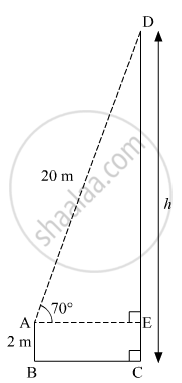Share

# A Ladder on the Platform of a Fire Brigade Van Can Be Elevated at an Angle of 70° to the Maximum. the Length of the Ladder Can Be Extended Upto 20 M. If the Platform is 2m Above the Ground - Geometry

ConceptHeights and Distances

#### Question

A ladder on the platform of a fire brigade van can be elevated at an angle of 70° to the maximum. The length of the ladder can be extended upto 20 m. If the platform is 2m above the ground, find the maximum height from the ground upto which the ladder can reach. (sin 70° = 0.94)

#### Solution

Suppose the maximum height from the ground upto which the ladder can reach be h m.Here, AB = 2 m, AD = 20 m, ∠DAE = 70º and AE ⊥ CD.
DE = CD − CE = (h − 2) m             (CE = AB)
In right ∆AED,
$\sin70^\circ = \frac{DE}{AD}$
$\Rightarrow 0 . 94 = \frac{h - 2}{20}$
$\Rightarrow h - 2 = 20 \times 0 . 94 = 18 . 8$
$\Rightarrow h = 18 . 8 + 2 = 20 . 8 m$

Thus, the maximum height from the ground upto which the ladder can reach is 20.8 m.

Is there an error in this question or solution?

#### APPEARS IN

Balbharati Solution for Balbharati Class 10 Mathematics 2 Geometry (2018 to Current)
Chapter 6: Trigonometry
Problem set 6 | Q: 9 | Page no. 139

#### Video TutorialsVIEW ALL 

Solution A Ladder on the Platform of a Fire Brigade Van Can Be Elevated at an Angle of 70° to the Maximum. the Length of the Ladder Can Be Extended Upto 20 M. If the Platform is 2m Above the Ground Concept: Heights and Distances.
S